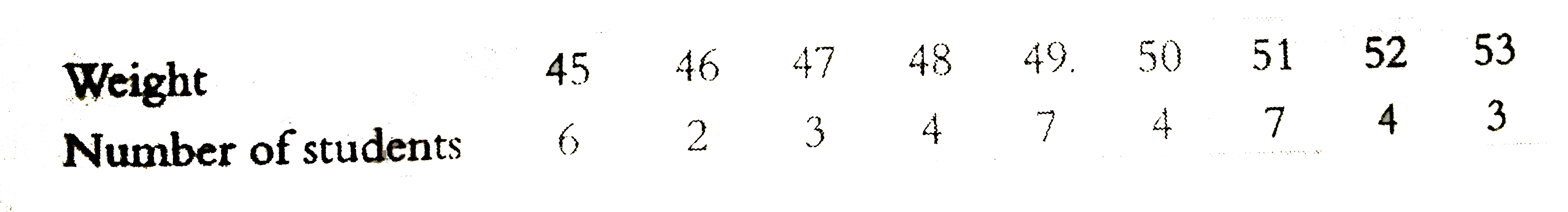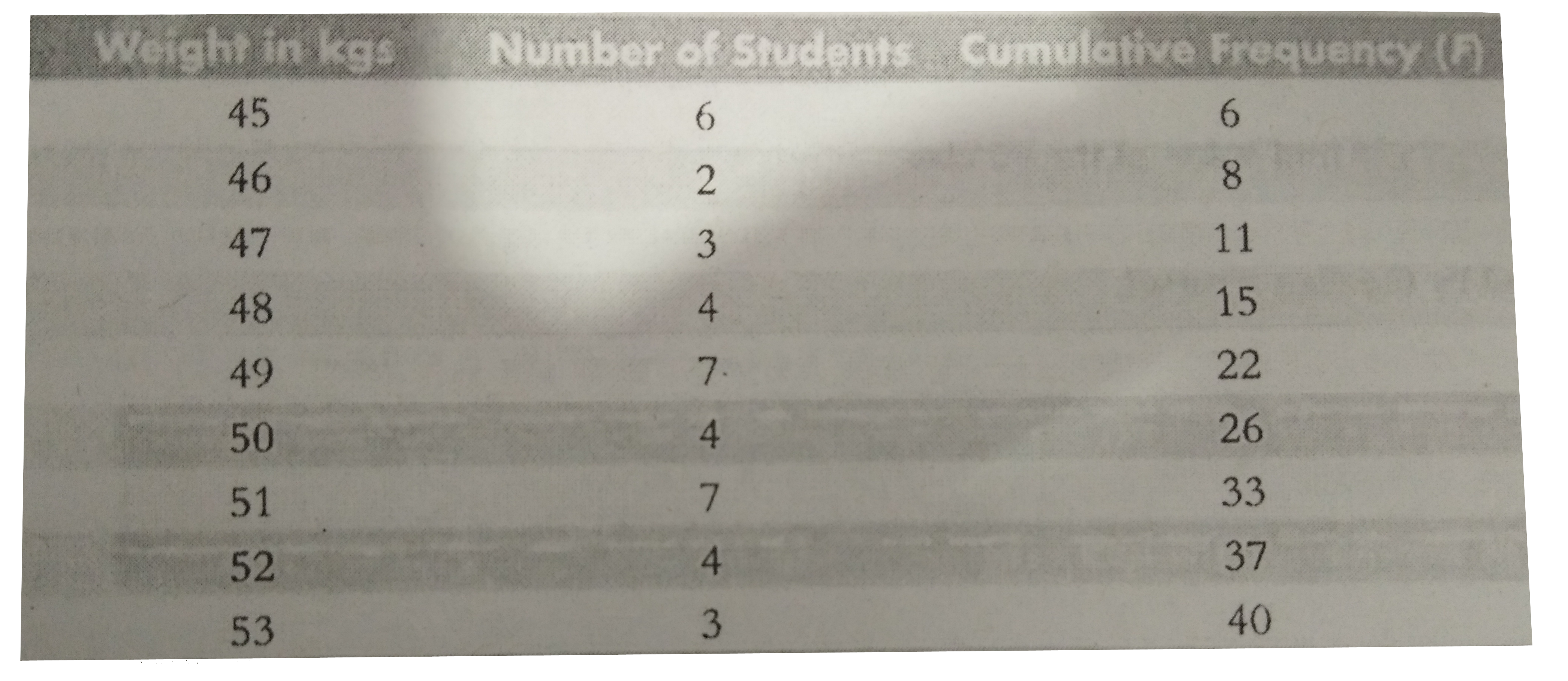# Following is the data showing weights of 40 students in a class . Find its median .

258 views

closed
Following is the data showing weights of 40 students in a class . Find its median .by (71.6k points)
selected by

To find the median , we prepare less than cumulative frequency table as given below :Here , n = 40 which is even .
therefore Median = Value of (40)/(2) , or the 20th observation .
From the column of cumulative frequency , the value of 20th observation is 49 .
therefore Median = 49 kg .